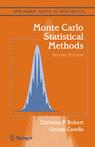## New typos in Monte Carlo Statistical MethodsThree weeks ago, I got this email from Amir Alipour, an Iranian student, about typos in Monte Carlo Statistical Method:

“I found some typos in the book which were not reported at your website. I list them blow, I would appreciate if you let me know if I`m right.
1.       Page 4, line 9,$(\theta_1,\ldots,\theta_n,p_1,\ldots,p_n)$, the index should not be$k$ instead of$n$?
2.       Page 4, example 1.3, last line,$n>q$, should be$n>=q$ (as we have$x_0$ ).
3.       Page 5, the likelihood of MA(q), it seems$\sigma^{-(n+q)}$ should  change to$\sigma^{-(n+q+1)}$.
4.       Page 8, formula (1.10).  The gradient symbol$\nabla$ is used for the first time without introducing, while it is used for the second time on page 19 with introducing.
5.       Page 8, Example 1.6, the log part in$\psi(\theta)$, should  change to$\log(-1/(2\theta_2))$.
6.       Page 10, in modified Bessel function,$z$ should change to$t$.
7.       Page 10, Example 1.9, in the likelihood function, the power of$\sigma$ should be$-n$, and the power of the function under product should be$-\frac{p+1}{2}$. (Even Figure 1.1 is not consistent with likelihood)”

and I have posted those new typos on the associated webpage. Amir Alipour has thus managed to find seven yet undiscovered typos in the first ten pages of the book! I am quite grateful to Amir Alipour for signaling those typos. Especially the final one which is due to an intented presentation of the$t$ density as a polynomial, with a poor wording: the likelihood of a$t$ sample is proportional to a power of a polynomial in the location parameter. (And there still is a typo since$\sigma^{n(p+1)/2}$ should be$\sigma^{2n/(p+1)}$…) Now I can only hope Amir Alipour can proceed through the whole book with the same amount of dedication!

### One Response to “New typos in Monte Carlo Statistical Methods”

1.More typos in Monte Carlo Statistical Methods « Xi'an's Og Says:

[…] typos in Monte Carlo Statistical Methods Following my earlier post, I received further typos from Amir Alipour and Gholamhussein Gholami, a former student of mine now […]

This site uses Akismet to reduce spam. Learn how your comment data is processed.